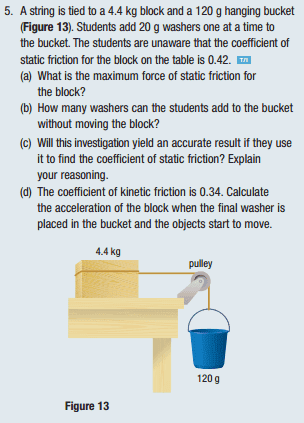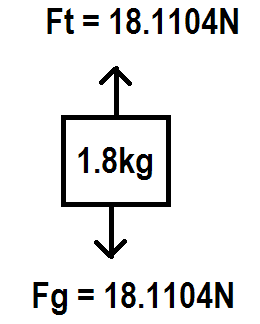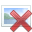# Tension with a pulley question

• AAAA

## Homework Statement## Homework Equations

us*Fn = Fs
FNET = ma
Fk = us*Fn

3. The Attempt at a Solution
A
0.42*9.8*4.4 = Fs
Fs = 18.1104N

B
F = ma
m = 18.1104N/9.8m/s/s
m = 1.848kg

1.848kg-0.120kg = 1.728kg for the washers to make up for

1.728kg/(0.02kg/washer) = ~86 washers

C
I'm not sure how to answer this question.

D
Fk = us*Fn
= 0.34*9.8*4.4
= 14.6608N

FNET = ma
Ft - Fk = (4.4+(1.848))*(a)
18.11 - 14.6608 = (4.4+(1.848))*(a)
a = 3.4496/(4.4+1.848)
a = ~0.552m/s/s

I get the right answers for this question, but what I don't understand is that, the force required to overcome static friction is ~18.11N. But the box is not getting the full 18.11N from the bucket, is it? Isn't some of that force used to push the bucket down, and then tension is what ends up needing to be 18.11N? How can the tension be the entire 18.1104N if some of that force is "used" to pull the bucket down?

Sorry if this is a little confusing. Thanks for any help.

Where do you think this down force is coming from?

Where do you think this down force is coming from?
From the bucket being accelerated by gravity.

But I think I get it now. The forces are balanced, nothing is moving, so that -Fg = Ft. The tension is equal to the force induced by gravity.

HANGING MASSAm I correct in my assumption?

I think your question/confusion is this:

If you pull on something using a rope, with a force, 18N say, and the resistance is 10N, then the object accelerates. But, you also think the tension in the rope should be 10N, not 18N.

The first point is that if the tension were only 10N, then why would the object accelerate? It would have no way to know that the force on the other end of the rope is 18N. It would only know about the 10N it feels. So, the tension must be 18N.

But, more fundamentally, I think you're not grasping the second law: F = ma. One of the consequences of this law is that if a force is not fully resisted by another force then it causes acceleration. I think you want the force in this case somehow to dissipate? You apply a force, the object accelerates and somehow the acceleration replaces the force? And the force is no longer measurable?

It's an interesting way to look at things! All I can say is that it's not correct. An unbalanced force that caused acceleration is still measurable. Both the acceleration and the force can be measured.

Concerning c: what happens if a student touches the experimental setup while putting one of the last washers in, or if the washer falls down the last centimeter and suddenly hits the bucket? Also consider your result from (D).

I think your question/confusion is this:

If you pull on something using a rope, with a force, 18N say, and the resistance is 10N, then the object accelerates. But, you also think the tension in the rope should be 10N, not 18N.

The first point is that if the tension were only 10N, then why would the object accelerate? It would have no way to know that the force on the other end of the rope is 18N. It would only know about the 10N it feels. So, the tension must be 18N.

But, more fundamentally, I think you're not grasping the second law: F = ma. One of the consequences of this law is that if a force is not fully resisted by another force then it causes acceleration. I think you want the force in this case somehow to dissipate? You apply a force, the object accelerates and somehow the acceleration replaces the force? And the force is no longer measurable?

It's an interesting way to look at things! All I can say is that it's not correct. An unbalanced force that caused acceleration is still measurable. Both the acceleration and the force can be measured.So is this the correct way of looking at this problem? ↓

The forces are balanced, nothing is moving, so that -Fg = Ft. The tension is equal to the force induced by gravity.So is this the correct way of looking at this problem? ↓

First, apologies, as I misunderstood what you were asking. So, ignore my first post.

If we are taking about part d), then no that's not correct. You don't need to consider the tension to solve the problem. If you do consider the tension, then you were right the first time. It will be less than the gravitational force on the bucket. For the reasons you explained!

I'm not sure what you don't understand. Do you want to calculate the tension in the rope for part d)?

PS I think I might have just understood what you're asking!

Last edited:
The diagram I made is regarding part a). What was confusing me is that some of the force must be "used" to pull the bucket down, and the rest is used as the tensile force to pull the cart. What I think now is that since the forces are balanced in part a) (like in the diagram), the force of tension must be 18.1104N, but later in part d), for example, there is a force imbalance which causes the bucket to accelerate (due to having a lower frictional force)→ in which case I think you do have less force than what is induced in the bucket by gravity, because some of the force is used to push it down.

Would this be correct for calculating tension in the bucket for part d)?
Fnet = ma
Ft - Fk = ma
Ft - 14.6608 = 4.4(0.552)
Ft - 14.6608 + 14.6608 = 2.4288 + 14.6608
Ft = 17.0896N of tension in the rope for part d)

So that means that 1.0208N of force are used to pull the bucket down, right?

1.0208N/1.848kg = 0.552m/s/s

Thank you all for your help so far.

The diagram I made is regarding part a). What was confusing me is that some of the force must be "used" to pull the bucket down, and the rest is used as the tensile force to pull the cart. What I think now is that since the forces are balanced in part a) (like in the diagram), the force of tension must be 18.1104N, but later in part d), for example, there is a force imbalance which causes the bucket to accelerate (due to having a lower frictional force)→ in which case I think you do have less force than what is induced in the bucket by gravity, because some of the force is used to push it down.

Would this be correct for calculating tension in the bucket for part d)?
Fnet = ma
Ft - Fk = ma
Ft - 14.6608 = 4.4(0.552)
Ft - 14.6608 + 14.6608 = 2.4288 + 14.6608
Ft = 17.0896N of tension in the rope for part d)

So that means that 1.0208N of force are used to pull the bucket down, right?

1.0208N/1.848kg = 0.552m/s/s

Thank you all for your help so far.

Yes. That sounds spot on to me.

I can't vouch for all your numbers, but that's definitely the right approach. It was good to double-check with the acceleration on both objects: that's a good habit to get into.

Yes. That sounds spot on to me.

I can't vouch for all your numbers, but that's definitely the right approach. It was good to double-check with the acceleration on both objects: that's a good habit to get into.
Alright, thanks a lot for all your help!